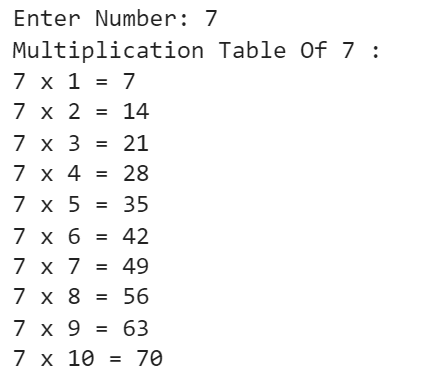# Create Multiplication Table In Python With Code Examples

Do you know what is multiplication table in Python? Have you ever thought about how to make multiplication table in Python? Let us try to understand.

We will try to understand python multiplication table with one real-life example. Why do we need to have a multiplication table Python? This will be cleared with one daily life example. Later we will try to provide a multiplication table example to clear the topic. Let us assume one daily life scenario to understand the Python multiplication table.

In our school days, when we all were primary or kindergarten students, we used to perform multiplication tables. There is no need to discuss what is multiplication tables & multiplication table example. As everybody knows what is it. It is a kind of numerical table. There for a particular number, a table produces the result of the multiplication with a series of numbers.

There is a multiplication table for each & every number. Now suppose, in your school, a weird question appears. You have to make a multiplication table of 43 number. As a primary student, it will be difficult for you at that time.

But now, it is not a difficult task for you. If you know the Python programming language, then it is a simple task for you to execute the task. For your help, there is the Python multiplication table. Multiplication table python helps you to generate the result of any multiplication table in one second.

But at that time, you can’t able to know the Python programming language. Even you don’t know what is a computer at that time. So, that time you might face problems. But now, if you have the Python multiplication table, then you can easily provide the answer. Now, if anybody asks you for the multiplication table of 334, then it will not be a difficult task for you. Simply you have to execute a multiplication table Python.

Here, we try to answer how to make multiplication table in Python. This will help to understand multiplication table example also. But before that, let us try to know what is Python programming language briefly.## What Is Python Programming Language? Read Below

Python is a smart-level programing language. It is so versatile that Python is being used by most corporates to develop new software. This programming language acts as a general-purpose programming language. It is not the programming language that follows the Object-Oriented Programming Language concept. It is completely a different programming language. Another great feature is that it takes a minimum number of lines to implement a code.

Python is attractive for its small amount of code consumption. For printing, any variable Python just consumes one line of code. On the other hand, other programming languages take more than five lines. Also, there is no need to include any header file. Python can able to perform the task automatically. Python is also used widely because it doesn’t take any semicolons for terminating any line. It only takes space as a terminating symbol.

## How To Make Multiplication Table In Python?

At first, we need to declare one integer variable in Python. This integer variable will responsible for taking user input. This means, here the users first provide the number. The number that is going to implement the multiplication table example. Creating a multiplication table in Python will be the most needed part of the code.

Then, there is no need to make any lengthy steps. At first, we need to run for a loop. Here we intend to make a multiplication table in Python that will run till the 10 number. So, the for loop will execute till the appearance of the 10 number. In each iteration of the for loop, the number is going to be multiplied by the variable. The variable will run from number 1 to number 10. It is a simple for-loop process to implement a multiplication table in Python.

Example:

```zap = int(input("Enter Number: ")) # Declaration Of The User Input Variable

print("Multiplication Table Of",zap,":") # Printing The Statement

for i in range(1, 11): # For Loop Iteration 10 Times From 1 To 10

print(zap, 'x', i, '=', zap*i) # Printing The Multiplication Table```

Let us try to find out the output of the above code. This will help to understand how we can create multiplication table in Python.

Output:## Conclusion:

As we saw multiplication table in Python is a very important topic.

We need to always remember how to make multiplication table in Python. This will help a lot in the future when we try to solve difficult problems.

It is advised to complete the basics of the Python programming language, which  will help to understand this topic in an easy & better manner. It is advised to practice Python programming language more & more.

So, hope you have liked this piece of article. Share your thoughts in the comments section and let us know if we can improve more.

DMCH also offers a wide range of programming and coding help services for you to benefit from. Don’t miss out! Visit domycodinghomework.com and our expert team will be there to help you out.